If you’re just starting off with Excel, and haven’t even taken a beginner’s course in how to use Excel, you may not be familiar with how spreadsheets work. At heart, a spreadsheet is a tool for organizing information and numbers, and performing calculations with those numbers. A spreadsheet presents information as a grid of rows and columns, with numbers for each row, and letters for each column. You put your information into the cells of the spreadsheet, and reference each cell based on the intersection between the row number and the column letter. A cell can contain many things, but usually it contains a number, a label, or a calculation such as adding a column of numbers.

Adding numbers is one of the most basic functions you perform in Excel. One thing you might try when you launch Excel for the first time is simply typing an equation into a cell, such as 2+2.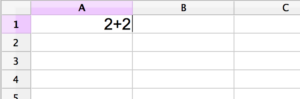If you try entering that into a cell right now, and hit return, you will see something like this.So what’s the problem? Isn’t Excel smart enough to add 2+2? Of course it is. The problem is that you haven’t figured out how to tell Excel that you want those numbers added. Right now Excel is looking at what you’ve put into this particular cell, and it interprets the symbols there as a label, not as a formula. In order to tell Excel that you want it to perform a calculation, you need to start the entry with an equal sign.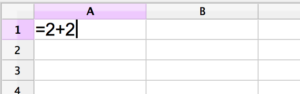If you enter “=2+2” into an Excel cell, and then hit return, the result you’ll get is this: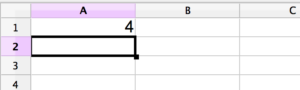Much better, right? Excel can perform simple calculations in any cell just by preceding them with an equal sign, and then typing in the mathematical formula for the calculation you desire.

But Excel wouldn’t be very useful if all that it could do was calculate numbers in a single cell. Instead of just putting in numbers, you can also put in the addresses of other cells. Remember how each cell is referenced by the relationship between the row and the column? Try putting two different numbers into different cells, and then in a third cell typing a formula to add them together. Don’t forget to start your formula with an equal sign. (To get the address of any cell, you can type out the letter for the column and then number for the row, one right after the other with no spaces.)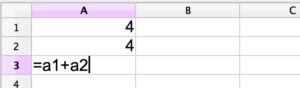If you put this in Excel then hit enter, you should see this: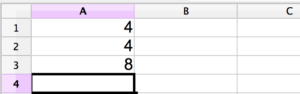See how Excel is taking the values in cell A1 and cell A2, and adding them together in cell A3? Excel’s ability to do this quickly and easily opens up a huge range of possibilities. The implications could fill an entire course of study on advanced Excel techniques.

Imagine that you had a column of five numbers, and you wanted to add them altogether. Can you think about how you would do that? Based on what we’ve seen so far, your first answer might look something like this: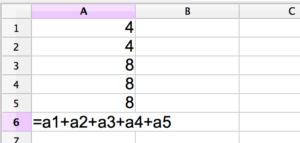If you entered that and hit return, you would see that Excel would correctly calculate the sum of all of the numbers in the column.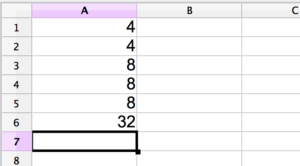But doing it this way would become very inconvenient if you had longer columns. Fortunately, Excel provides a shortcut to let you tell it to add together any series of cells that are contiguous. To do this, you use the Excel function sum(). In the parentheses you pass Excel the address of the first cell, a colon, and then the address of the last cell. This syntax tells Excel that you mean the entire range, from the first cell to the last cell, including everything in between.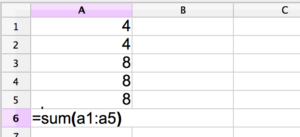This gives you the same result as putting in each one of the cell addresses individually, but it’s much easier to type. In fact, summing together contiguous cells of numbers is such a common operation that Excel provides a couple of useful shortcuts to make it easier to do.

## Excel Shortcuts

The first one is clicking. In order to enter the address of a specific cell into a formula you’re writing, all you have to do is click that cell, and the address will be added automatically. So for example, if you were entering a sum() formula and got to the point where you needed the very first cell of the range, all you would have to do is click on the cell you wanted included in the formula while you’re typing, and that address would be entered for you.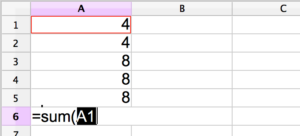Excel also allows you to select a range of cells by clicking and dragging from the first cell to the last cell, in any order.Another convenient shortcut is the sum symbol. You may have seen this mathematical symbol, represented by the Greek letter sigma (∑), which is used to stand for the concept of summing values.Adding a column of numbers is such a common thing to do in Excel that at the top of the interface you will often see a sigma to let you do that more easily. All you have to do is click in an empty cell at the bottom of a column of numbers you want to add together, and then hit the sigma symbol in the interface. Excel will automatically enter a formula for calculating the sum of all of the numbers in that column above the empty cell.

Of course, the convenience of being able to add contiguous numbers isn’t the only thing that Excel is good at. Excel provides a wide range of functions, and using these shortcuts allows you to pass cells, ranges of cells, and even noncontiguous sets of cells to those functions easily.

If you think about the implications of this, you can understand why Excel is such an important and powerful tool for businesses. To learn more about it, you can start with a beginners class in Excel, and then move on to a more advanced class in Excel. You’ll be amazed at the power you can command with this complex piece of software.

Page Last Updated: January 2014

### Top courses in Excel

Microsoft Excel: Data Analysis with Excel Pivot Tables
Maven Analytics, Chris Dutton
4.7 (57,280)
Bestseller
Microsoft Excel Pro Tips: Go from Beginner to Advanced Excel
Maven Analytics, Chris Dutton
4.7 (5,147)
Bestseller
Microsoft Excel for Construction Management
James Gedney-Higham
4.4 (483)
Bestseller
Microsoft Excel - Excel from Beginner to Advanced
Kyle Pew, Office Newb
4.7 (410,252)
Bestseller
Microsoft Excel: Data Visualization, Excel Charts & Graphs
Maven Analytics, Chris Dutton
4.6 (44,436)
Bestseller
Microsoft Excel - Excel from Beginner to Advanced 2023
Warrick Klimaytys
4.6 (9,279)
Bestseller